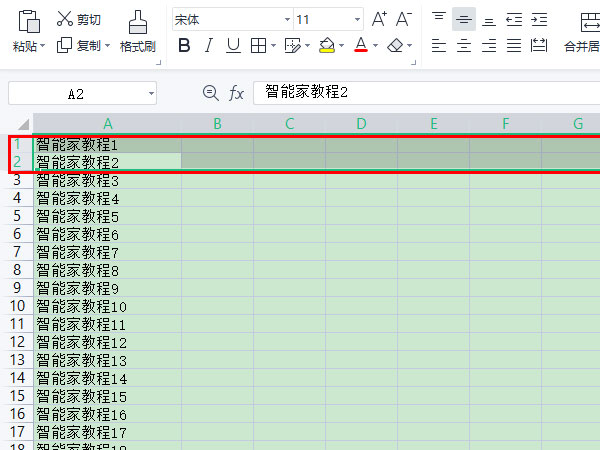# 获取Excel表格列序号和列名的方法

﻿在处理Excel文件时，通常要获取xx列的数据，这就要求先找到列序号（从0开始）。### 1、常量和进制转换方法

```//列名元素集
const COL_ABC = ["A", "B", "C", "D", "E", "F", "G", "H", "I", "J", "K", "L", "M", "N", "O", "P", "Q", "R", "S", "T", "U", "V", "W", "X", "Y", "Z" ];

/**
* 十进制的数化为k进制的数【返回数组的index=0位置是最高位】（取k取余法）
* @num 10进制数
* @k k
* @returns k进制数序列
*/
function ckqy(num,k) {
let y = [];
while (num > 0) {
let s = Math.floor(num / k);
let _y = num % k;
//console.log(num + " ÷ " + k + " ＝ " + s + " ··· " + _y);
if (s == 0)
{
y.push(num);
num = 0;
}
else
{
y.push(_y);
num = s;
}
}
return y.reverse();
}

/**
* k进制的数化为十进制的数(乘幂相加法)
* @kArray k进制数序列
* @k k
* @returns 十进制的数
*/
function kTo10(kArray,k){
let v10=0;
let kArrayLen=kArray.length;
for(let ind=0; ind<kArrayLen; ind++){
v10 += kArray[ind] * Math.pow(k,kArrayLen - 1 - ind);
}
return v10;
}

```

### 2、获取列名

```/**
* 获取列名
* @colIndex 列序号（从0开始）
* @returns 列名
*/
function getColName(colIndex)
{
if(colIndex < 0){
return '';
}
let colNames =[];
// 等于0 和 大于25 的 colIndex
let isAdd1 = colIndex > 25 || colIndex < 1 ;
//10进制转26进制
let kArray = ckqy(colIndex + (isAdd1 ? 1: 0), 26);
for (let i = 0; i < kArray.length; i++)
{
colNames.push(COL_ABC[ kArray[i]- (isAdd1 ? 1: 0) ] || '');
}
return colNames.join('');
}
```

### 3、获取列序号

```/**
* 获取列名对应ABC数据序号
* @colIndex 列序号（从0开始）
* @returns
*/
function getABCIndex(colName){
let kArray = [];
let colNames=colName.split('');
for(let ind=0;ind <colNames.length;ind++){
let k = COL_ABC.indexOf(colNames[ind]);
if(k > -1){
kArray.push(k);
}
}
return kArray;
}
/**
* 获取列序号
* @colIndex 列名
* @returns 列序号（从0开始）
*/
function getColIndex(colName)
{
if(!colName){
return -1;
}
// colName 转 COL_ABC 序号
let kArray = getABCIndex(colName);
let kArrayLen=kArray.length;
if(kArrayLen==0){
return -1;
}
let colIndex =0;
for(let ind=0;ind <kArrayLen;ind++){
let k = kArray[ind] + (  (kArrayLen-1)  == ind ? 0:1);
let p =  kArrayLen-1-ind;
//console.log(k+' * Math.pow(26,'+p+');');
colIndex += k * Math.pow(26,p);
}
return colIndex;
}
```

### 4、使用

```let _colName = 'ABC';
let colIndex=getColIndex(_colName);
console.log(colIndex);
let colName = getColName(colIndex);
console.log(colName);
console.log(_colName  == colName  );
```

## 相关文章

•获取Excel表格列序号和列名的方法

这篇文章主要介绍了获取Excel表格的列序号和列名的方法，当中将涉及到10进制和k进制的互转，需要的小伙伴可以参考一下，希望对你有所帮助
2021-12-02
•excel如何固定第一和第二行?excel冻结第一第二行方法

excel如何固定第一和第二行?下面小编就来带大家具体的操作方法吧，不妨一起看看吧
2021-11-28
•如何在Microsoft Excel 中创建3D地图

如何在 Microsoft Excel 中创建 3D 地图？今天小编就为大家带来了详细的在MicrosoftExcel 中创建 3D 地图，感兴趣的朋友一起看看吧
2021-10-14
•2021-10-11
•Excel怎么使用MINVERSE函数计算矩阵的逆矩阵?Excel计算逆矩阵教程

Excel中有很多的函数小伙伴们都不会使用，小伙伴们对MINVERSE函数有没有了解呢？他可以计算出矩阵的逆矩阵，一起来看看如何使用
2021-09-28
•excel表格怎么做一个简单的成绩查询系统?

excel表格怎么做一个简单的成绩查询系统？学校都有成绩查询系统，用于考试后查询成绩的，该怎么制作一个简单的成绩查询系统呢？下面我们就来看看详细的教程
2021-09-27
•excel表格怎么修改图表里的系列1系列2? excel图表标题修改方法

excel表格怎么修改图表里的系列1系列2？excel表格中创建的图表添加了系列1和系列2，该怎么修改系列呢？下面我们就来看看excel图标标题修改方法
2021-09-22
•Excel中如何使用NORMSINV函数?Excel使用NORMSINV函数教程

NORMINV函数是用于返回指定平均值和标准偏差的正态累积分布函数的反函数值，那么大家知道Excel中怎么使用NORMSINV函数吗？跟着小编一起往下看看吧
2021-09-15
•Excel怎么设计销售漏斗图?Excel设计销售漏斗图教程

销售漏斗图是用来衡量网站中业务流程表现，适用于电商等各个行业，漏斗图可以非常直观地看出网站业务流程中的问题所在，那么如何使用Excel绘制销售漏斗图呢
2021-09-15
•如何在excel表格单元格中间插入一条线? excel表格单元格中间添加线条的

Excel是我们经常使用的办公软件，excel表格单元格中间怎么添加线条？今天小编将为大家带来的是excel表格单元格中间怎么添加线条的方法，有需要的朋友赶紧来看看
2021-09-11